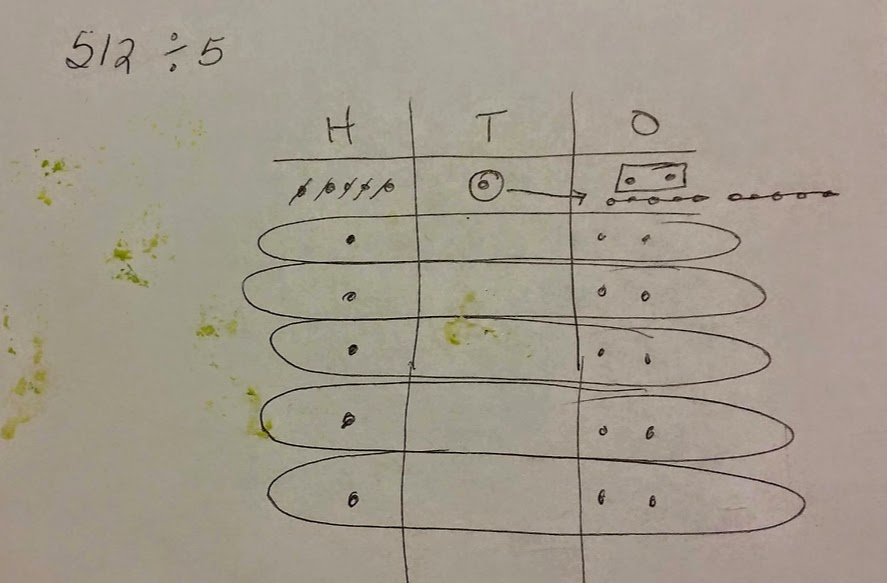# Place Value Chart to Divide Hundreds and Thousands

23 teachers like this lesson
Print Lesson

## Objective

SWBAT find whole number quotients and remainders using a place value chart.

#### Big Idea

In this lesson, students divide whole numbers by a one digit divisor, decomposing tens, hundreds, and thousands, to find a whole number quotients and remainders.

## Warm Up

5 minutes
Because basic facts are so important for my students to master, and more than half of my class does not have them mastered, I chose an oral division facts practice for this warm up.  I change the PowerPoint slides every 3 seconds. I encourage my students to yell their answers "loud and proud."

Being able to multiply and divide fluently within 100 is essential prior to fourth grade, and the basic facts of addition subtraction and multiplication/division are a critical baseline.  Students must be fluent in these skills in order to be successful with later work with fractions, decimals, ratios, and proportion. As students begin to learn math facts, their brains are focused on those basic computations, but as students become automatic with basic facts, their brains are then able to focus on other aspects of the task like the challenges of place value, decimals, or fractions. Being automatic with basic facts frees the brain to focus on other math processes. Committing basic math facts to memory speeds up math tasks.

## Concept development

40 minutes

This lesson is the third lesson in a series of lesson about using a place value chart to divide. To see the first lesson click here, and to see the second lesson, click here.

I chose to do this lesson series to give students a solid understanding of distribution as a way to divide. Before Common Core standards, teachers may have given a premature introduction to the standard division algorithm.  This does little to promote student understanding of the operation or of the meaning behind computational procedures. In classrooms where rote memorization of algorithmic steps is emphasized students often make computational errors without understanding why they are doing so.

I begin this lesson by showing a division problem on the board like 178 divided by 4.  I model the problem on the board, using a place value chart, as students model the problem in their math notebooks.  In today's lesson, students build and extend their learning from the previous lessons to solve division problems with dividends in the thousands and hundreds with remainders. Students will need to decompose thousands, hundreds, and tens to be successful with this lesson.  In the problem set students work on, I also include problems with a zero in the dividend and quotient,  and model with students how to record the quotient.

I give students 8 problems to work on during the class period.  Students that did not finish took it home as homework.  The problems students worked on in class and then took home were:

3248 / 3

1986 / 5

486 / 8

521 / 5

97 / 7

129 / 3

242 / 4

75 / 4

You can see one of the above problems worked out in this photo. Click here to hear more information about decomposing hundreds and thousands.You can also click here to see a student's math notebook and my thoughts about this student's work and the division progression.

Just as I had done in the previous lessons, I am explicit with my division vocabulary and use correct place value names. I also show how this model connects to the long division algorithm. Students check their work with multiplication.  (This is also a way for me to observe students multiplication skills and see who has not yet mastered this.)

The learning activities provided in this series of lessons give students opportunities to represent mathematical ideas using pictures, diagrams, numbers, and symbols.  Representing ideas in a variety of ways helps students to model and interpret problem situations, understand mathematical concepts, clarify and communicate their thinking, and make connections between related mathematical ideas. Students’ own concrete and pictorial representations of mathematical ideas provide me with valuable assessment information about student understanding that I often cannot assess effectively using paper-and-pencil tests.  Common Core State Standards require students to be able to think deeply and make connections between mathematical ideas and concepts.  Common Core Standard 4.NBT.6 asks that students use strategies based on place value and the relationship between multiplication and division to find whole number quotients.  Since initial experiences with division in the primary grades, and third grade, often involve sharing objects equally, this series of lessons involving a place value chart to divide builds upon students prior understanding, while incorporating place value strategies as stated in the standard.